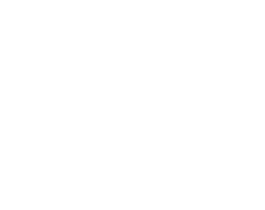Aptitude Questions: Mensuration Set 9

Hello Aspirants. Welcome to Online Quantitative Aptitude section in AffairsCloud.com. Here we are creating question sample from Mensuration that are important for all the competitive exams. We have included some questions that are repeatedly asked in exams !!

1. A rectangular box has dimensions 1.6 m × 1 m × 0.6 m. How many cubical boxes each of side 20 cm can be fit inside the rectangular box?
A) 220
B) 205
C) 120
D) 165
E) 124
C) 120
Explanation:

20 cm = 0.2 m
So number of boxes that can fit = 1.6 × 1 × 0.6/0.2 × 0.2 × 0.2

2. What is the volume of a cylinder whose curved surface area is 704 cm2 and height is 14 cm?
A) 2878 cm3
B) 2914 cm3
C) 2396 cm3
D) 2816 cm3
E) 2916 cm3
D) 2816 cm3
Explanation:

2ᴨrh = 704, h = 14
Solve both, so r = 8
Volume = ᴨr2h

3. If the volume of wire remains the same but its radius decreases to 1/3rd of previous, then the new length of the wire will be how many times of the previous length?
A) 3 times
B) 4 times
C) 6 times
D) 10 times
E) 9 times
E) 9 times
Explanation:

Wire will be taken as cylinder.
R = r/3
ᴨr2h = ᴨR2H
r2h = (r/3)2H
so H = 9h

4. A cone with diameter of its base as 12 cm is formed by melting a spherical ball of diameter 6 cm. What is the height of the cone?
A) 6 cm
B) 3 cm
C) 32 m
D) 15 cm
E) None of these
B) 3 cm
Explanation:

Radius of cone = 12/2 = 6, radius of ball = 6/2 = 3
Volumes will be equal, so
(1/3)ᴨ r2h = (4/3)ᴨ R3
62h = 4* 33
So h = 3

5. The total cost of painting the walls of a room is Rs 475. Find the cost of painting the walls of another room whose length, breadth and height each are double than the dimensions of the previous room.
A) Rs 1780
B) Rs 1900
C) Rs 1846
D) Rs 1960
E) Rs 1600
B) Rs 1900
Explanation:

Area of first room = 2(l+b)*h
After all dimensions doubled, new area = 2(2l+2b)*2h = 4[2(l+b)*h ] = 4 times previous area, so cost of painting = 4*475

6. A circular wire of diameter 84 cm is bent into a rectangle with sides ratio 6 : 5. What are the respective sides of the rectangle?
A) 60 cm, 72 cm
B) 78 cm, 65 cm
C) 72 cm, 60 cm
D) 90 cm, 75 cm
E) None of these
C) 72 cm, 60 cm
Explanation:

Length of wire = 2ᴨr = 2(22/7)*42 = 264 cm
Perimeter of rectangle = 2(6x+5x) = 264
Solve, x = 12
So dimensions – 12*6, 12*5

7. The ratio of the outer and the inner perimeters of a circular path is 9 : 8. The path is 3 metres wide. What is the diameter of the outer circle?
A) 20 m
B) 27 m
C) 35 m
D) 39 m
E) 24 m
B) 27 m cm3
Explanation:

2ᴨr/2ᴨR = 9/8
So r/R = 9/8, so r = (9/8)R
r-R = 3, so (9/8)R – R = 3
R = 24, so r = (9/8)*24 = 27

8. Four circular cardboard pieces, each of diameter 28 cm are placed in such a way that each piece touches two other pieces. Find the area of the space enclosed by the four pieces.
A) 195 cm2
B) 134 cm2
C) 168 cm2
D) 162 cm2
E) None of these
C) 168 cm2
Explanation:Required area = area of square shown in figure – 4*areas of 1/4th parts of each circle, so
Required area = 28*28 – 4*(1/4)*(22/7)*14*14

9. A solid sphere of radius 20 cm is melted to form 12 spherical balls. Find the radius of each of the ball.
A) 12 cm
B) 8 cm
C) 20 cm
D) 10 cm
E) 15 cm
D) 10 cm
Explanation:

Volume of each of the 8 ball = [(4/3)*(22/7)*203]/8
So (4/3)*(22/7)*r3 = [(4/3)*(22/7)*203]/8
Solve, r = 10

10. The perimeter of a rectangular plot is 210 m. Find the cost of gardening 2 m broad boundary around rectangular plot whose perimeter The cost of gardening is Rs 14 per m2.
A) Rs 6450
B) Rs 6400
C) Rs 6480
D) Rs 6104
E) Rs 6880
D) Rs 6104
Explanation:

Given 2(l+b) = 210
2 m broad boundary means increase in l and b by 4 m
So area of the boundary will be [(l+4)(b+4) – lb] = 4(l+b) + 16 = 2*[2(l+b)] + 16 = 2*210 + 16 = 436 m2
So cost of gardening = 436*14

AffairsCloud Recommends Oliveboard Mock Test

AffairsCloud Ebook - Support Us to Grow

error: Alert: Content is protected !!